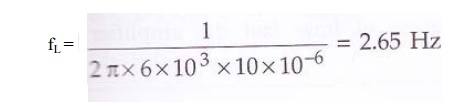Home | | Electronic Circuits I | Sag and its Relation to Lower Cut-off Frequency

# Sag and its Relation to Lower Cut-off Frequency

The amplifier low frequency RC network consists of coupling and bypass capacitors make amplifier output to decrease with large time constant.

Sag and its Relation to Lower Cut-off Frequency:

The amplifier low frequency RC network consists of coupling and bypass capacitors make amplifier output to decrease with large time constant. As a result, the output voltage has sag or tilt associated with it as shown in the following figure.The tilt or sag in time t1 is given by,The lower 3 dB frequency can be determined from the output response by carefully measuring the tilt.

The lower 3 dB frequency is given asSo, the lower 3 dB frequency can be represented in terms of tilt is measured from the following figureProblem 1:

For a circuit shown in the following figure, calculate percentage tilt. Assume approximate h-parameter circuit for the transistor.Equivalent h-parameter circuit for the given circuit is,Here R1 = RC + RL = 4K + 2K = 6KΩWe know that, P = (∏fL / f) * 100

Assuming f = 200 Hz

P = (∏ * 2.65 / 200) * 100

P = 4.1%

Study Material, Lecturing Notes, Assignment, Reference, Wiki description explanation, brief detail
Electronic Circuits : Frequency Analysis of BJT and MOSFET Amplifiers : Sag and its Relation to Lower Cut-off Frequency |